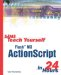# String Operations and Functions

 I l @ ve RuBoard

Now that we can get strings from the user , it will be useful to manipulate them.

#### Concatenation

To combine two strings, use the + symbol. For instance, if you have a variable named myVariable and want to add something to it, just use myVariable + and the thing you want to add. Here is an example using the Output window:

` var myVariable = "Hello"; trace(myVariable+" World."); `

The resulting output will be "Hello World." The variable still contains just "Hello" , but the trace command gets the full "Hello World."

You can also add the string to the end of the variable permanently using += :

` var myVariable = "Hello"; myVariable += " World."; trace(myVariable); `

You can combine the + symbol in all sorts of ways. Here is another example:

` var myVar1 = "This"; var myVar2 = "and"; var myVar3 = "that"; var myVar4 = myVar1 + " " + myVar2 + " " + myVar3; trace(myVar4); `

The resulting output is "This and that."

#### Substrings

You can also get parts of a string. For instance, if you wanted to get the fourth to seventh letters of a string, you could so this:

` var myString = "Hello World."; trace(myString.substring(3,7)); `

The result would be "lo W." But the numbers in the function need some explaining. The function got the fourth to seventh characters , but why was the function fed a 3 and a 7 instead of a 4 and a 7?

Unfortunately, the explanation is a little confusing. First, remember that in ActionScript, counting usually starts at 0. So the first character of the string is character 0. That means that the fourth character is 3. So that explains the 3. But if the first parameter is 3, then wouldn't the second parameter be 6? No, because the second parameter is actually the number of the character to stop before. So if you want to stop before character 6, you need to use a 7.

Therefore to get the fourth through seventh characters, we use a 3 and a 7. Just remember to subtract 1 at all times when picking out character placement, but that the second parameter of substring needs to have 1 added to it.

There is actually another way to get parts of strings. I think you will like this one better. The substr function has a similar name but works differently. Its two parameters are the start character and the length of the substring needed. So you can get four characters, starting at character 3, with this:

` var myString = "Hello World."; trace(myString.substr(3,4)); `

The result is the same as the previous example ”"lo W."

One more command that you should know is charAt . You can use this to get a single character from the string. For instance, this gets character 6, the "W," from the string.

` var myString = "Hello World."; trace(myString.charAt(6)); `

#### String Functions

There are a few miscellaneous string functions that you may want to have in your arsenal of ActionScript functions. You can use indexOf to find the position of a string inside another string. For instance, to find the "W," you could do this:

` var myString = "Hello World."; trace(myString.indexOf("W",0)); `

This returns a 6, because "W" is the sixth character. You can also find strings longer than one character:

` var myString = "Hello World."; trace(myString.indexOf("llo",0)); `

This returns a 2, because the string "llo" starts at character 2. Note that the 0 as the second parameter of these two functions means that the search starts at that point. A 0 starts the search at the beginning of the string. If we used a 2, the search would ignore the first two characters and start at the third character.

If the indexOf function doesn't find the search string, it returns a -1.

You can use lastIndexOf to find a string starting at the back of the other string. For instance, to find the first "l" and the last "l," you can do this:

` var myString = "Hello World."; trace(myString.indexOf("l",0)); trace(myString.lastIndexOf("l")); `

The results are a 2 and a 9. The 2 represents the first "l" in "Hello," and the 9 represents the "l" in "World."

ActionScript also has useful functions that convert all the letters in a string to uppercase or lowercase. Here is an example:

` var myString = "Hello World."; trace(myString.toUpperCase()); trace(myString.toLowerCase()); `

The results in the Output window look like this:

` HELLO WORLD. hello world. `

One more thing you should know about strings is how to get their length. For this, just use the length property of the string. Here is an example:

` var myString = "Hello World."; trace(myString.length); `

The answer is 12. Remember that just because we start counting characters at 0 doesn't affect how many characters there are. So the first character is character 0, the last is character 11, and the length is 12.

With a good working knowledge of how to manipulate strings, you can create any number of interesting applications in Flash. A simple one would be a user input form, just like the ones used on the Web that are usually in HTML.

 I l @ ve RuBoardSams Teach Yourself Flash MX ActionScript in 24 Hours
ISBN: 0672323850
EAN: 2147483647
Year: 2002
Pages: 272